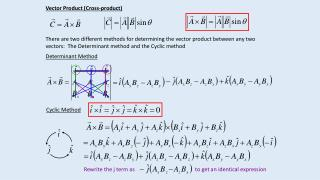DownloadDownload PresentationVector Product (Cross-product)

# Vector Product (Cross-product)

Download Presentation## Vector Product (Cross-product)

- - - - - - - - - - - - - - - - - - - - - - - - - - - E N D - - - - - - - - - - - - - - - - - - - - - - - - - - -
##### Presentation Transcript

1. Vector Product (Cross-product) There are two different methods for determining the vector product between any two vectors: The Determinant method and the Cyclic method Determinant Method Cyclic Method Rewrite the j term as to get an identical expression

2. The direction of the resultant vector, for a right-handed coordinate system, for a vector product can be determined using the right-hand rule. Fingers point in the direction of the second vector The vector product looks at the product of two vectors which are perpendicular to each other, and who are also perpendicular to the resultant vector. Thumb points in the direction of first vector. Palm points in the direction of resultant vector. Example: Determine the magnitude and direction of the area of a parallelogram described by the vectors r1 and r2 which are used to describe the length of the two sides. y r1 r2 x Out of the page

3. Torque When a force is applied to an object it will cause it to accelerate. This acceleration would correspond to a change in the velocity of the object. The velocity could be a translational velocity or a rotational velocity. A torque is a force that causes a change in the angular velocity of the object. The force F1 will cause a counterclockwise rotation about an axis that passes through O. The force F2 will cause a clockwise rotation about an axis that passes through O. r is the distance from the origin O to the point of application of F. q1 r1 d is the lever arm for F which is the distance from O to F such that d is perpendicular to F. q2 r2 t – Torque [Nm] r – distance from axis of rotation [m] F – Force [N] q – angle measured from r to F d = rsinq this is the lever arm, the component of r perpendicular to F The direction of the torque vector is perpendicular to both r and F.The directions clockwise and counterclockwise are used to describe the direction the torque causes the object to rotate.

4. Each force applied to an object can cause that object to rotate. If the object is initially at rest and then begins to rotate due to a torque, the object must have undergone an angular acceleration. Compare to This is Newton’s second law written for rotational motion. The net torque on an object will cause that object to undergo an angular acceleration. For a single particle: For rigid object: Assume at is constant